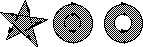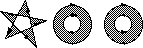## 4.3 Fill Rules

Two different approaches can be used to specify what is inside a shape: even-odd fill rule and (non-)zero winding fill rule. The following examples illustrate the two approaches.

Figure 7: Fill Rules

Non-Zero Winding rule   Even-odd ruleThe non-zero winding rule determines whether a given point is inside a shape by (conceptually) drawing a ray from that point to infinity in any direction and then examine the places where a segment of the shape crosses the ray. Starting with a count of zero, the count is incremented each time a segment crosses the ray from left to right and decremented each time a segment crosses from right to left . After counting all the crossings, if the result is zero then the point is outside the shape, otherwise it is inside. With this rule, a simple convex shape yields inside and outside as would be expected.

Now consider a five pointed star, drawn with five connected straight line segments intersecting each other. The entire area enclosed by the star, including the pentagon in the center, is considered inside by the non-zero winding rule. For a shape composed of two concentric circles, if they are both drawn in the same direction, the areas enclosed by both circles are inside according to the rule. If they are drawn in opposite directions, only the area between the two circles is inside according to the rule; the 'hole' is outside.

The even-odd rule determines whether a given point is inside by drawing a ray from that point in an arbitrary direction and counting the number of segments that the ray crosses. If the number is odd the point is inside; if even, the point is outside. The even-odd rule yields the same results as the non-zero winding rule for simple shapes, but different results for more complex ones. For the five pointed star drawn with five intersecting lines, the even-odd rule considers the triangular parts to be inside, but the pentagon in the center to be outside. For the two concentric circles, only the area between the two circles is inside, regardless of the directions of the circles.

The non-zero winding rule is more versatile than the even-odd rule and is the default rule used by Bifrost to determine what is inside and outside of a shape. Since the even-odd rule is occasionally useful for special effects or for compatibility with other graphics systems, optionally, this rule may be used instead.

 The rule does not specify what to do if a segment coincides with or is tangent to the ray. Since any ray will do, one may simply choose a different ray that does not encounter such problem intersections

 The Bifrost Graphics System - Reference Manual © 1991-2004 Mjølner Informatics [Modified: Tuesday October 24th 2000 at 15:02]